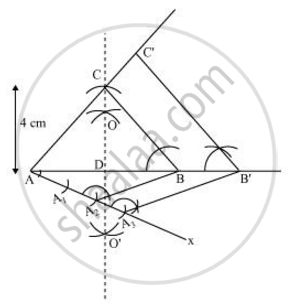# Construct an isosceles triangle whose base is 8 cm and altitude 4 cm and then another triangle whose side are 1 (1/ 2) times the corresponding sides of the isosceles triangle. Give the justification of the construction - Mathematics

Construct an isosceles triangle whose base is 8 cm and altitude 4 cm and then another triangle whose side are 1 1/2 times the corresponding sides of the isosceles triangle.

Give the justification of the construction

#### Solution

Let us assume that ΔABC is an isosceles triangle having CA and CB of equal lengths, base AB of 8 cm, and AD is the altitude of 4 cm.

A ΔAB'C' whose sides are 3/2 times of ΔABC can be drawn as follows.

Step 1

Draw a line segment AB of 8 cm. Draw arcs of same radius on both sides of the line segment while taking point A and B as its centre. Let these arcs intersect each other at O and O'. Join OO'. Let OO' intersect AB at D.

Step 2

Taking D as centre, draw an arc of 4 cm radius which cuts the extended line segment OO' at point C. An isosceles ΔABC is formed, having CD (altitude) as 4 cm and AB (base) as 8 cm.

Step 3

Draw a ray AX making an acute angle with line segment AB on the opposite side of vertex C.

Step 4

Locate 3 points (as 3 is greater between 3 and 2) A1, A2, and A3 on AX such that AA1 = A1A2 = A2A3.

Step 5

Join BA2 and draw a line through A3 parallel to BA2 to intersect extended line segment AB at point B'.

Step 6

Draw a line through B' parallel to BC intersecting the extended line segment AC at C'. ΔAB'C' is the required triangle.Justification

The construction can be justified by proving that

AB' = 3/2 AB, B'C' = 3/2 BC, AC' = 3/2 AC

In ΔABC and ΔAB'C',

∠ABC = ∠AB'C' (Corresponding angles)

∠BAC = ∠B'AC' (Common)

∴ ΔABC ∼ ΔAB'C' (AA similarity criterion)

=> (AB)/(AB')= (BC)/(B'C') = (AC)/(AC') ....(1)

In ΔAA2B and ΔAA3B',

∠A2AB = ∠A3AB' (Common)

∠AA2B = ∠AA3B' (Corresponding angles)

∴ ΔAA2B ∼ ΔAA3B' (AA similarity criterion)

=> (AB)/(AB') = (

=>(AB)/(AB') = 2/3    .....2

On comparing equations (1) and (2), we obtain

(AB)/(AB')=(BC)/(B'C') = (AC)/(AC') = 2/3

=> AB' = 3/2 AB, B'C' =  3/2 BC, AC' = 3/2 AC

This justifies the construction.

Concept: Division of a Line Segment
Is there an error in this question or solution?
Chapter 11: Constructions - Exercise 11.1 [Page 220]

#### APPEARS IN

NCERT Class 10 Maths
Chapter 11 Constructions
Exercise 11.1 | Q 4 | Page 220

Share